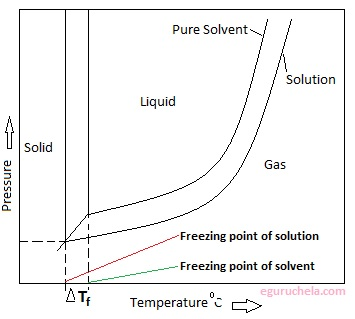# Depression of freezing point

Freezing-point depression describes the process in which adding a solute to a solvent decreases the freezing point of the solvent. Examples include salt in water, alcohol in water, or the mixing of two solids such as impurities in a finely powdered drug. In the last case, the added compound is the solute, and the original solid is thought of as the solvent. the phenomenon that occurs when the freezing point of a liquid (a solvent) is lowered by adding another compound to it, such that the solution has a lower freezing point than the pure solvent.

### Example:

The freezing point of seawater or even ordinary saltwater is lower than the freezing point of pure water.

Freezing point depression can be calculated using the Clausius-Clapeyron equation and Raoult's law. In a dilute ideal solution the freezing point is

Freezing Pointtotal = Freezing Pointsolvent - ΔTf

where ΔTf = molality * Kf * i

Kf = cryoscopic constant (1.86°C kg/mol for the freezing point of water) i = Van't Hoff factor

The freezing points of solutions are all lower than that of the pure solvent. It is directly proportional to the molality of the solute.

#### ΔTf = Tf (solvent) - Tf(solution) = Kf x m

where

ΔTf is the freezing point depression,

Tf (solvent) is the freezing point of the solvent,

Tf(solution) is the freezing point of the solution,

Kf is the freezing point depression constant and

m is the molality### Uses of freezing point depression

To lower the freezing point of water, sodium chloride is spread over the roads in the areas having lower temperatures. This helps in preventing the build-up of ice over the roads.

Calcium chloride is used instead of NaCl in places with temperatures below 18 0C to melt the ice on the roads, because of the fact that calcium chloride dissociates into three ions. This causes greater depression in the freezing point of water.

In automobiles Ethylene glycol and water are generally used to make radiator fluids which helps in preventing the freezing of the radiator during the winter season.

The formula of freezing point depression can be used to:

-Estimate the degree to which a solute can dissociate in a solvent.

-Determine the molar mass of a given solute.